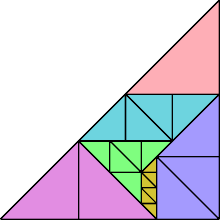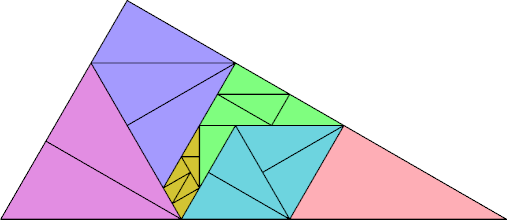# Contiguous Reverse Partridge Tilings

## Introduction

This formula holds for every number n:

Σ(i=1; n) i3 = T(n)2,

where T(n) is the nth triangular number. This formula implies that a square with side T(n) might be dissected into 1 square of side 1, 2 squares of side 2, and so on up to n squares of side n. Such a tiling is called a partridge tiling, after the partridge in the song The Twelve Days of Christmas.

Robert Wainwright was the first to propose partridge tilings, and the first to find one: a square of side T(12), dissected in partridge fashion into squares with sides 1 through 12. Partridge tilings were later extended to shapes other than squares.

A reverse partridge tiling is a dissection in which the number of tiles decreases as the scale of the tile increases. For some values of n there is a number k such that

k2 = Σ(i=1; n) i2(n+1−i).

The first two values of n for which k is an integer are 6 (k=14) and 25 (k=195). Many polygons are known to have reverse partridge tilings for k=14, and a few for k=195. You can see them at Erich Friedman's Math Magic page for June 2007. They include solutions for the square, the equilateral triangle (and hence any triangle), the triamond, the L tetromino, and the monodrafter.

Here I show all known reverse partridge tilings with n=6 in which equal tiles are contiguous. According to Wainwright, this subproblem has apparently not been studied before. If you solve any cases not shown here, please write.

## Polyominoes

The monomino, or square, has two contiguous solutions:By transforming these solutions one may obtain a solution for any rectangle or parallelogram.

## Polytans

The moniamond, or equilateral triangle, has no contiguous solution with 6 scales. If it did, such a solution could be distorted to solve other triangles such as the monotan, the monodrafter, and the monodom.

The smallest solution for the monotan, or monabolo, uses sizes 1 through 4 to make a monotan at scale 5√2. This format has no contiguous solutions. But with sizes 1 through 6 there are many solutions. Here is one:The right trapezoidal tritan also has many solutions.The parallelogrammatic ditan has a solution with n=4:## Polyiamonds

The triamond has many solutions:## Polydrafters

The monodrafter has at least one solution:## Polydoms

The monodom has several solutions.This parallelogrammatic didom and tetradom can be solved with fewer scales than the monomino:Last revised 2021-06-02.
Back to Polyform Tiling < Polyform Curiosities
Col. George Sicherman [ HOME | MAIL ]